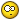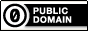Jump to content
• Sign Up

## Recommended Posts

Wow Melba.. thank you so very much! Fantastic code edit there and you also took care of the Hash issue also. I'll pipe the result to the cfg file using that. hehe.. Yes it seems easy to you Autoit coding wizs but I'm still getting the hang of this. Utterly brilliant work there, Melba! Really happy about this now. So much appreciated to the community for their support!

#### Share this post

##### Share on other sites

Thudo,

Here is how I might go about it:```#include <Crypt.au3>
#include <File.au3>

#cs <<<<<<<<<<<<<<<<<<<<<<<<<<<<<<<<<<<<<<<<<<<<<<<<<<<<<<<<<<<<<<<<<<<<<<<<<<<<<<<<<<<<<<<<<<<<<<<<<<<
; Determine cfg paths
Local \$TempDir=("\\<network_path>\")        ; network path to cfg
Local \$TempDir2=("C:\DATA\")                ; local path to cfg (used as backup)

; Choose alternate path if primary does not exist
If Not FileExists(\$TempDir) then
Local \$sav = \$TempDir2 & '\ScreenSaver.cfg', \$lticks, \$GUI, \$CGUI, \$bl_ScreenSaver = False
Local \$sav = \$TempDir2 & '\ScreenSaver.cfg', \$lticks
Local \$w = @DesktopWidth, \$h = @DesktopHeight, \$sPath = FileReadLine(\$sav, 1)
Else
Local \$sav = \$TempDir & '\ScreenSaver.cfg', \$lticks, \$GUI, \$CGUI, \$bl_ScreenSaver = False
Local \$sav = \$TempDir & '\ScreenSaver.cfg', \$lticks
Local \$w = @DesktopWidth, \$h = @DesktopHeight, \$sPath = FileReadLine(\$sav, 1)
endif
#ce <<<<<<<<<<<<<<<<<<<<<<<<<<<<<<<<<<<<<<<<<<<<<<<<<<<<<<<<<<<<<<<<<<<<<<<<<<<<<<<<<<<<<<<<<<<<<<<<<<<<

Opt('GUIOnEventMode', 1)

#Region Pref GUI
\$PGUI = GUICreate('Screensaver Utility', 280, 150, -1, -1, BitOR(0x00040000, 0x00000080))
GUISetOnEvent(-3, '_PrefClose')

GUICtrlCreateGroup('Configuration Folder', 5, 5, 245, 42)
;\$p_pic = GUICtrlCreateEdit(FileReadLine(\$sav, 1), 10, 20, 170, 20, 2048 + 128)
\$p_pic = GUICtrlCreateEdit("Folder", 10, 20, 170, 20, 2048 + 128)
GUICtrlCreateButton('Browse', 190, 20, 50, 20)
GUICtrlSetOnEvent(-1, '_Browse')

GUICtrlCreateGroup('Idle timeout', 170, 50, 80, 40)
;\$tidle = GUICtrlCreateEdit(FileReadLine(\$sav, 4), 180, 67, 60, 17, 8192 + 128)
\$tidle = GUICtrlCreateEdit("Idle", 180, 67, 60, 17, 8192 + 128)

GUICtrlCreateGroup('Enter new Password', 5, 50, 155, 40)
;~ \$pass = GUICtrlCreateEdit(FileReadLine(\$sav, 5), 10, 65, 145, 20, 8192 + 128)    ; Displays existing MD5 Hash in the cfg file
;\$pass = GUICtrlCreateEdit("", 10, 65, 145, 20, 8192 + 128)
\$pass = GUICtrlCreateInput("", 10, 65, 145, 20, 0x0020) ; \$ES_PASSWORD style <<<<<<<<<<<<<<<<<<<<<<<<<<<<<<<<<<<<<<<<<<<<<<<<<<<<<<<<<<<<<<<

GUICtrlCreateButton('Apply', 80, 100, 80, 20)
GUICtrlSetOnEvent(-1, '_PrefApply')
GUISetState(@SW_HIDE, \$PGUI)
#EndRegion Pref GUI

; Always run the application
_PrefOpen()

#Region Pref Func
Func _Browse()
\$path = FileSelectFolder('Select configuration folder', "", 1 + 2)
If @error Then Exit ConsoleWrite('!> FileSelectFolder' & @CRLF)
GUICtrlSetData(\$p_pic, \$path & '\')
EndFunc   ;==>_Browse

Func _PrefApply()

Local \$iMinLength = 8, \$iDigits = 1, \$iLowerCase = 0, \$iUpperCase = 1, \$iSpecialChars = 0

; <<<<<<<<<<<<<<<<<<<<<<<<<<<<<<<<<<<<<<<<<<<<<<<
; Read Password input
\$sPassWord = GUICtrlRead(\$pass)
; Check complexity
If _CheckPasswordComplexity(\$sPassWord, \$iMinLength, \$iDigits, \$iLowerCase, \$iUpperCase, \$iSpecialChars) = 0 Then
\$Error = "Password does not conform to the rules, it must have: "
If \$iMinLength > 0 Then \$Error &= "a minimum length of " & \$iMinLength & " Characters, "
If \$iDigits > 0 Then \$Error &= "at least " & \$iDigits & " Numbers, "
If \$iLowerCase > 0 Then \$Error &= \$iLowerCase & " or more lowercase characters, "
If \$iUpperCase > 0 Then \$Error &= "no less than " & \$iUpperCase & " Uppercase characters, "
If \$iSpecialChars > 0 Then \$Error &= "at least " & \$iSpecialChars & " Special characters such as punctuation, "
\$Error &= "Please change your password appropriately."
MsgBox(48, "Error", \$Error)
GUICtrlSetData(\$pass, "")
Return
EndIf
; So if we get here, the password is good - so now we hash it
_Crypt_Startup()
\$sPassWord_Encrypted = _Crypt_HashData(\$sPassWord, \$CALG_MD5)
_Crypt_Shutdown()

; And to show the result:
MsgBox(0, "Result", "Password = " & \$sPassWord & @CRLF & "Hash = " & \$sPassWord_Encrypted)

#cs <<<<<<<<<<<<<<<<<<<<<<<<<<<<<<<<<<<<<<<<<<<<<<<<<<<<<<<<<<<<<<<<<<<<<<<<<<<<<<<<<<<<<<<<<<<<<<<<<<<<

_FileWriteToLine(\$sav, 1, GUICtrlRead(\$p_pic), 1)   ; Write the new path location to the cfg
If (GUICtrlRead(\$tidle) <> '') Then _FileWriteToLine(\$sav, 4, GUICtrlRead(\$tidle), 1)   ; Write the new timeout # to the cfg
If (GUICtrlRead(\$tidle) = '') Then _FileWriteToLine(\$sav, 4, GUICtrlRead(\$tidle), 1)    ; Write the new timeout # to the cfg
;~  If (GUICtrlRead(\$pass) <> '') Then FileWrite(\$sav, 5)   ; Write the new password converted to MD5 Hash to the cfg (in progress)
;~  If (GUICtrlRead(\$pass) = '') Then FileWrite(\$sav, 5)    ; Write the new password converted to MD5 Hash to the cfg (in progress)

Return Call('_PrefClose')

#ce <<<<<<<<<<<<<<<<<<<<<<<<<<<<<<<<<<<<<<<<<<<<<<<<<<<<<<<<<<<<<<<<<<<<<<<<<<<<<<<<<<<<<<<<<<<<<<<<<<<<

EndFunc   ;==>_PrefApply

Func _PrefOpen()

#cs <<<<<<<<<<<<<<<<<<<<<<<<<<<<<<<<<<<<<<<<<<<<<<<<<<<<<<<<<<<<<<<<<<<<<<<<<<<<<<<<<<<<<<<<<<<<<<<<<<<<

GUICtrlSetData(\$p_pic, FileReadLine(\$sav, 1))
GUICtrlSetData(\$tidle, FileReadLine(\$sav, 4))
;~  GUICtrlSetData(\$pass, FileReadLine(\$sav, 5))    ; Displays existing MD5 Hash in the cfg file

#ce <<<<<<<<<<<<<<<<<<<<<<<<<<<<<<<<<<<<<<<<<<<<<<<<<<<<<<<<<<<<<<<<<<<<<<<<<<<<<<<<<<<<<<<<<<<<<<<<<<<<

GUISetState(@SW_SHOW, \$PGUI)
EndFunc   ;==>_PrefOpen

Func _PrefClose()
Exit GUISetState(@SW_HIDE, \$PGUI)
EndFunc   ;==>_PrefClose
#EndRegion Pref Func

While 1
Sleep(10)
WEnd

Func _CheckPasswordComplexity(\$sPassword, \$iMinLength, \$iDigits = 0, \$iLowerCase = 0, \$iUpperCase = 0, \$iSpecialChars = 0)

Local \$sRegExp = "^.*(?=.{" & \$iMinLength & ",})"
If \$iDigits > 0 Then \$sRegExp &= "(?=.*[[:digit:]]{" & \$iDigits & ",})"
If \$iLowerCase > 0 Then \$sRegExp &= "(?=.*[[:lower:]]{" & \$iLowerCase & ",})"
If \$iUpperCase > 0 Then \$sRegExp &= "(?=.*[[:upper:]]{" & \$iUpperCase & ",})"
If \$iSpecialChars > 0 Then \$sRegExp &= "(?=.*[[:punct:]]{" & \$iSpecialChars & ",})"

\$sRegExp &= ".*\$"

Local \$iResult = StringRegExp(\$sPassword, \$sRegExp)
If @error = 0 Then
Return SetError(0, 0, (\$iResult <> 0))
Else
Return SetError(1, 0, 0)
EndIf

EndFunc   ;==>_CheckPasswordComplexity```

I have had to comment out a lot of your original code - look for the #ce/s <<<<<<<<<<< lines.

I also changed the password edit into an input as it is better suited.

Not so difficult really was it?M23Any of my own code posted anywhere on the forum is available for use by others without any restriction of any kind

Open spoiler to see my UDFs:

Spoiler

ArrayMultiColSort ---- Sort arrays on multiple columns
ChooseFileFolder ---- Single and multiple selections from specified path treeview listing
Date_Time_Convert -- Easily convert date/time formats, including the language used
ExtMsgBox --------- A highly customisable replacement for MsgBox
GUIExtender -------- Extend and retract multiple sections within a GUI
GUIFrame ---------- Subdivide GUIs into many adjustable frames
GUIListViewEx ------- Insert, delete, move, drag, sort, edit and colour ListView items
GUITreeViewEx ------ Check/clear parent and child checkboxes in a TreeView
Marquee ----------- Scrolling tickertape GUIs
NoFocusLines ------- Remove the dotted focus lines from buttons, sliders, radios and checkboxes
Notify ------------- Small notifications on the edge of the display
Scrollbars ----------Automatically sized scrollbars with a single command
StringSize ---------- Automatically size controls to fit text
Toast -------------- Small GUIs which pop out of the notification area

#### Share this post

##### Share on other sites

Thudo,

Glad we could help.M23Any of my own code posted anywhere on the forum is available for use by others without any restriction of any kind

Open spoiler to see my UDFs:

Spoiler

ArrayMultiColSort ---- Sort arrays on multiple columns
ChooseFileFolder ---- Single and multiple selections from specified path treeview listing
Date_Time_Convert -- Easily convert date/time formats, including the language used
ExtMsgBox --------- A highly customisable replacement for MsgBox
GUIExtender -------- Extend and retract multiple sections within a GUI
GUIFrame ---------- Subdivide GUIs into many adjustable frames
GUIListViewEx ------- Insert, delete, move, drag, sort, edit and colour ListView items
GUITreeViewEx ------ Check/clear parent and child checkboxes in a TreeView
Marquee ----------- Scrolling tickertape GUIs
NoFocusLines ------- Remove the dotted focus lines from buttons, sliders, radios and checkboxes
Notify ------------- Small notifications on the edge of the display
Scrollbars ----------Automatically sized scrollbars with a single command
StringSize ---------- Automatically size controls to fit text
Toast -------------- Small GUIs which pop out of the notification area

#### Share this post

##### Share on other sites

Hey Melba.. I could sure use your help examining the end of this thread HERE. I've got this working, in theory, as a single script but when merging it as a GUI is not so easy. Thank you so very much! Really do appreciate your involvement here. MouseTrap can be a real challenge to get working when needed.

#### Share this post

##### Share on other sites

Hey Melba one other thing.. for the Min Timeout (to limit users to a minimum value of 5) I've attempted the following:

```Local \$iMinTime = 5, \$iValue = 0

; <<<<<<<<<<<<<<<<<<<<<<<<<<<<<<<<<<<<<<<<<<<<<<<
; Read Timeout input
\$tidle = GUICtrlRead(\$timeidle)
; Check value
If _CheckMinimumIdleTime(\$tidle, \$iMinTime, \$iValue) = 0 Then
\$Error = "Timeout must be no less than 5 minutes: "
If \$iMinTime > 0 Then \$Error &= "a minimum length of " & \$iMinTime & " Characters, "
;~         If \$iValue > 0 Then \$Error &= "Must be at least " & \$iMinTime & " mins, "
\$Error &= "Please change the time."
MsgBox(48, "Error", \$Error)
GUICtrlSetData(\$tidle, "")
Return
EndIf```

and

```Func _CheckMinimumIdleTime(\$tidle, \$iMinTime, \$iValue)

Local \$sRegExp = "^.*(?=.{" & \$iMinTime & ",})"
If \$iValue > 0 Then \$sRegExp &= "(?=.*[[:value:]]{" & \$iValue & ",})"

\$sRegExp &= ".*\$"

Local \$iResult = StringRegExp(\$tidle, \$sRegExp)
If @error = 0 Then
Return SetError(0, 0, (\$iResult <> 0))
Else
Return SetError(1, 0, 0)
EndIf

EndFunc   ;==>_CheckMinimumIdleTime```
However not working out as expected. Basically trying to get the user when they input a value under 5 in the GUICtrlRead(\$timeidle) field to warn them the value must be greater than 5. So the timeouts must be always greater than 5 so if they put in a 2 it warns them it must be no less than 5.

Thanks so much for your patience.#### Share this post

##### Share on other sites

Can anyone help with the above? In a pickle here.#### Share this post

##### Share on other sites

No worries.. its been solved via this thread!

Edited by Thudo

## Create an account or sign in to comment

You need to be a member in order to leave a comment

## Create an account

Sign up for a new account in our community. It's easy!

Register a new account

## Sign in

Already have an account? Sign in here.

Sign In Now

• ### Recently Browsing   0 members

No registered users viewing this page.

×

• Wiki

• Back

• #### Beta

• Git
• FAQ
• Our Picks
×
• Create New...